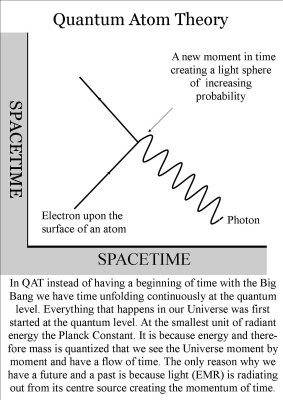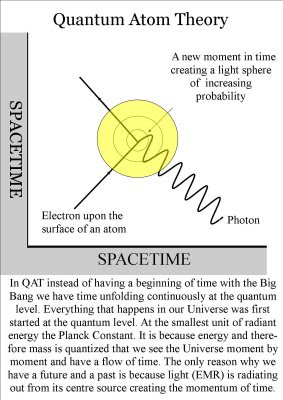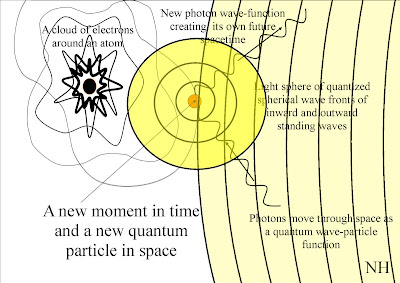Tuesday, 26 May 2009

Quantum Electrodynamics and the problem of renormalization.

QED and the problem of infinities
~In QED there is a problem the problem can only be put right in a process called renormalization. This is a mathematical problem the calculations for each coupling on a Feynman diagram are infinite. These infinities can only be canceled out by renormalization.

~In Quantum Atom Theory we have these infinities not because there is something wrong with the mathematics of QED but because we have no fundamental understanding of time. Sir Isaac Newton thought time existed as a ‘thing in itself’ and that time is intimately connected to motion. But if time exists in itself there must also be a process creating new moments in time that are also moments of infinity.

~Each coupling on a Feynman diagram represents such a moment in time and this is why we have the infinities that have to be canceled out by renormalization.
~From each photon electron coupling EMR will radiates out in a light sphere of quantized spherical wave fronts. Each wave front will be quantized at the level of the Planck Constant.

~The mathematical infinities of QED represent the infinity of our expanding Universe in space and time.

~When these wave fronts come in contact with electrons on the surface of other atom the wave function will collapse into a new quantum particle. In the form of a photon that will have its own position in time and space.
~There is then a quantum leap of energy creating a new wave function of future possibilities. This is a continuous process within our universe that Einstein called stimulated emissions that creates a chain reaction of photon electron couplings. Put very simply the light emitted by an atom now is going to be absorbed by other atoms later on and this is the fundamental process that creates the time continuum or arrow of time.
~The centre of each expanding light sphere represents a new moment in time. The larger the light sphere the greater the probability of any future event. This means that all the possible events in the Universe can be analysable into separate quantum events of which everything is a compound.

~

Friday, 1 May 2009

Infinity found in Beauty and sound

~

In Quantum Atom Theory time is being created continuously at the quantum level in a very simple process. When light waves of electromagnetic radiation comes in contact with the electrons on the surface of an atom. There will be a photon electron coupling and the wave function will collapse creating a new quantum particle in space and a new moment in time. There is then a quantum leap of energy creating a new wave function of future possibilities. We have Cantors’ continuum hypothesis because creation is being created continuously at the quantum level. But an explanation of infinity would be nothing without an explanation of beauty.
~

The other day I found Ray Tomes’ Harmonic Theory in this theory all waves in three dimensional Space will vibrate or oscillate creating harmonics. These harmonics will continuously resonate creating harmonic fractions of themselves in cycles and similarities of ever greater diversity. Over the great time scales of our Universe this will lead to the evolution of the fractal self-similarities that we see all around us today. Because these natural harmonics mimic music and have fundamental symmetry we have a Universe full of beauty in which each part is a fractal self-similarity of the whole, creating its own time and space.
~

Harmonic Theory and Q.A.T are very similar and are both trying to explain different aspects of the same thing. One the wave structure of matter the other the time continuum. An understanding of time can explain how we can have finite harmonic standing waves within infinite space. The Electromagnetic Spectrum is infinite therefore our Universe must an infinity. But just like a violin string which is bound at both ends the wave function or standing wave will be bound at both ends by a moment in time in the form of a quantum particle. In this way each moment in time creates its own harmonics. I believe that light waves of electromagnetic radiation are the medium for the propagation of these harmonic waves. Each moment in time is created by a photon electron coupling creating the smallest amount of radiant energy the Planck Constant. In this way time itself is quantized into the momentum of spacetime.
~Light sphere of standing waves.

~